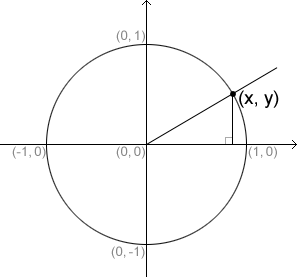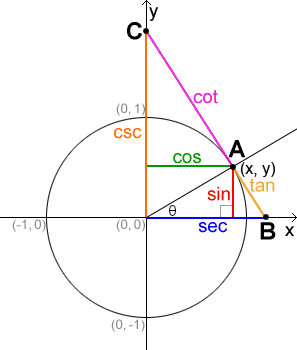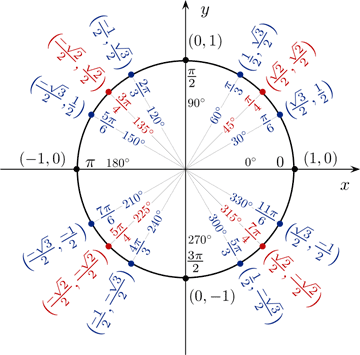# Unit Circle

A unit circle is a circle with radius 1 centered at the origin of the rectangular coordinate system. It is commonly used in the context of trigonometry.When a ray is drawn from the origin of the unit circle, it will intersect the unit circle at a point (x, y) and form a right triangle with the x-axis, as shown above. The hypotenuse of the right triangle is equal to the radius of the unit circle, so it will always be 1. Based on the Pythagorean Theorem, the equation of the unit circle is therefore:

x2 + y2 = 1

This is true for all points on the unit circle, not just those in the first quadrant, and is useful for defining the trigonometric functions in terms of the unit circle.

## Unit circle definitions of trigonometric functions

The unit circle is often used in the definition of trigonometric functions. Below is a figure showing all of the trigonometric relationships as they relate to the unit circle.In the figure above, point A has coordinates of (x, y). Together with θ, the angle formed between the initial side of an angle along the positive x-axis and the terminal side of the angle formed by rotating the ray counter-clockwise, we can form a right triangle. Using the fact that the radius of the unit circle is 1 (and therefore the hypotenuse of the right triangle is equal to 1), we can use the right triangle definitions of the trigonometric functions to find that, and. Based on this, we can determine the definitions of the rest of the trigonometric functions, as shown in the table below.

Trigonometric functions## Commonly used angles

While we can find trigonometric values for any angle, some angles are worth remembering because of how frequently they are used in trigonometry. The angles are 30°, 45° and, 60°. In radians, they correspond torespectively. Below is a table of the values of these angles, as well as a figure of the values on a unit circle.

Anglesin(θ)cos(θ)tan(θ)1As can be seen from the table or the unit circle above, there are three values to remember:. Because of the nature of the unit circle, these values are the same for their respective angles in different quadrants on the unit circle, with the only difference being their signs based on the quadrant the angle is in. Therefore, remembering these three values and how they correspond to multiples of 30°, 45° and 60° will enable you to fill in all the values on the unit circle.

The other angles on the unit circle to remember are those whose terminal sides lie on the x- or y-axis: 0° or 0 (which has equivalent sine and cosine values as 360° or 2π), 90° or, 180° or π and, 270° or. At any of these angles, sin(θ) or cos(θ) has a value of –1, 0, or 1.

Anglesin(θ)cos(θ)tan(θ)01010Undefined
180° or π0-10-10Undefined

## Method for memorizing common values

One method that may help with memorizing the common trigonometric values is to express all the values of sin(θ) as fractions involving a square root. Starting from 0° and progressing through 90°, sin(0°) = 0 =. The subsequent values, sin(30°), sin(45°), sin(60°), and sin(90°) follow a pattern such that, using the value of sin(0°) as a reference, to find the values of sine for the subsequent angles, we simply increase the number under the radical sign in the numerator by 1, as shown below.

 θ 0° 30° 45° 60° 90° sin(θ)The values of sine from 0° through -90° follows the same pattern except that the values are negative instead of positive since sine is negative in quadrant IV. This pattern repeats periodically for the respective angle measurements, and we can identify the values of sin(θ) based on the position of θ in the unit circle, taking the sign of sine into consideration: sine is positive in quadrants I and II and negative in quadrants III and IV.

A similar memorization method can be used for cosine. Starting from 0° and progressing through 90°, cos⁡(0°)=1=. The subsequent values, cos(30°), cos(45°), cos(60°), and cos(90°) follow a pattern such that, using the value of cos(0°) as a reference, to find the values of cosine for the subsequent angles, we simply decrease the number under the radical sign in the numerator by 1, as shown below:

 θ 0° 30° 45° 60° 90° cos(θ)From 90° to 180°, we increase the number under the radical by 1 instead, but also must take into account the quadrant that the angle is in. Cosine is negative in quadrants II and III, so the values will be equal but negative. In quadrants I and IV, the values will be positive. This pattern repeats periodically for the respective angle measurements.

As long as we remember these values, it is possible to use the relationship